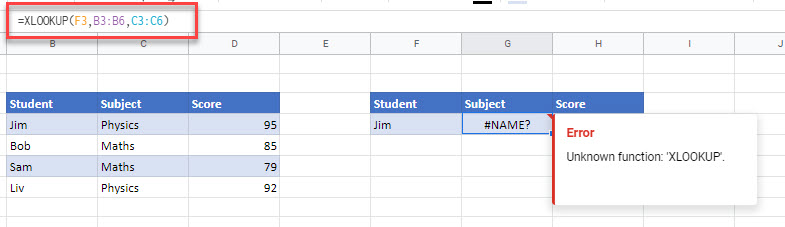# How to Copy & Paste Formulas Between Excel & Google Sheets

Last updated on February 15, 2023

This tutorial demonstrates how to copy and paste formulas between Excel and Google Sheets.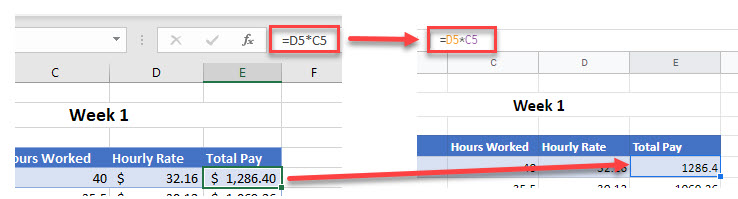## Copy Formulas

### Show Formulas

You can copy data and formulas from Excel to Google Sheets by making the formulas visible in Excel, and then copy-pasting the data into your Google sheet.

1. In Excel, press CTRL + ` (back quote) on the keyboard to make your formulas visible on the screen.
Find the back quote on the same key as the tilde (~), directly below ESC.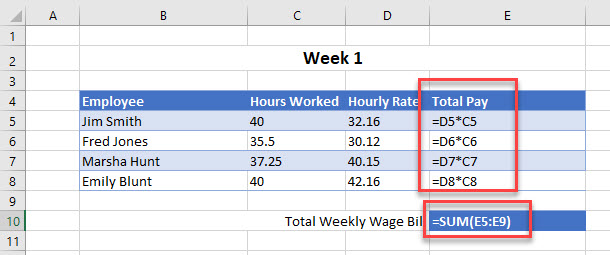1. Then, in the Ribbon, go to Home > Clipboard > Copy to copy the data (including the formulas).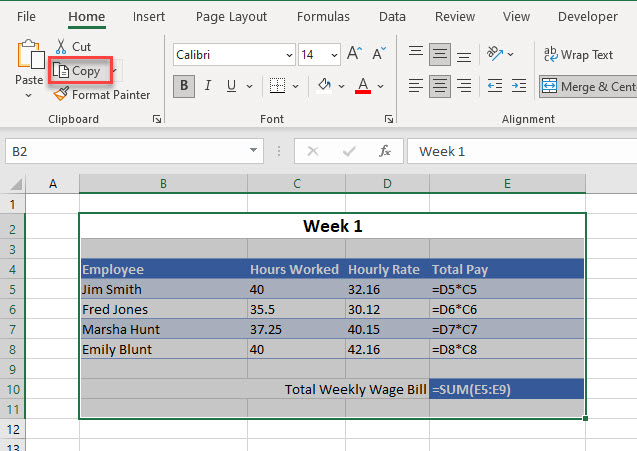1. Switch to your Google sheet, click where you wish your data to be pasted, and then press CTRL + V on the keyboard to paste the data and formulas. Notice that any cells that contained formulas in Excel are pasted into the Google sheet with their formulas.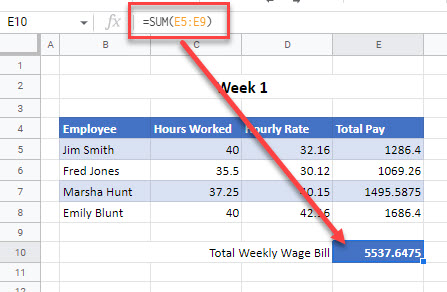### Find and Replace

Another method you can use to copy formulas is to use Find and Replace.

1. In Excel, select the cells that contain the formulas you wish to copy, and then in the Ribbon, go to Home > Find & Replace > Replace.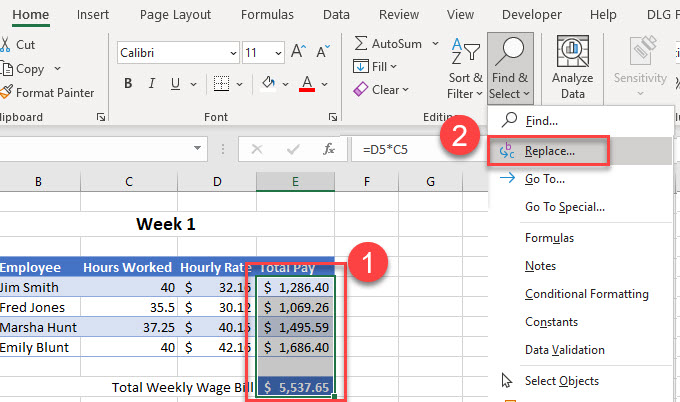1. In the Find and Replace dialog box, under Find what, enter the symbol you chose in Step 3, and under Replace with, enter = (an equal sign). Then click Replace All.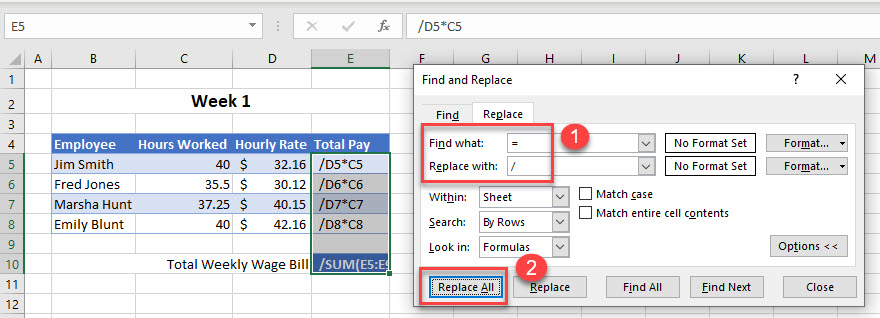1. Now, copy the selection and switch to your Google sheet and then press CTRL + V to paste the formulas where you need them to go in your Google sheet.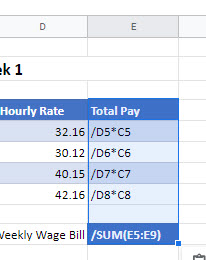1. Then, in the Menu, go to Edit > Find and Replace, or press CTRL + H.
In the Find box, type in a / (front slash) symbol and then type a = (equals) symbol in the Replace with box. Finally, click Replace all.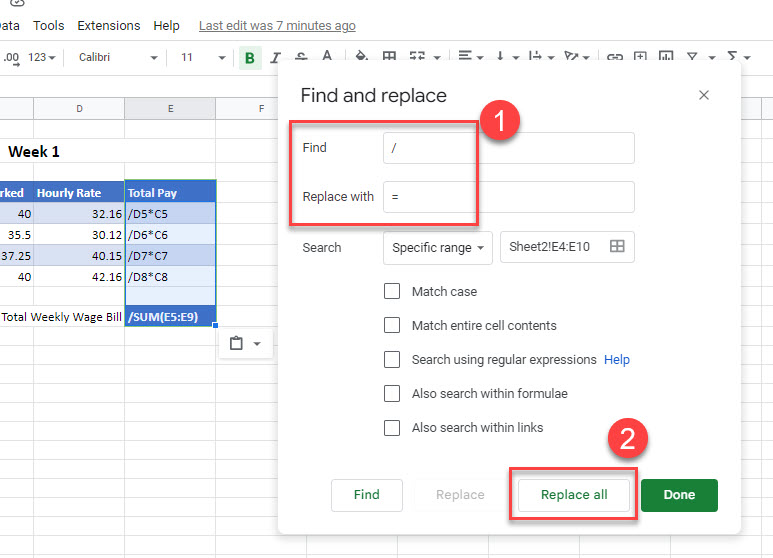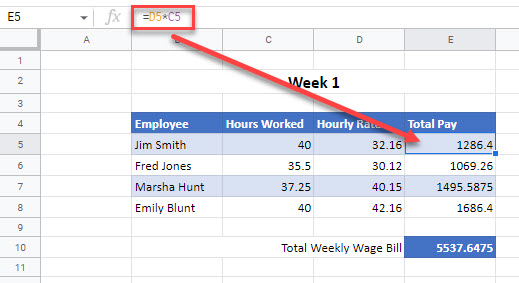## Which Formulas Can’t I Copy?

You can follow the steps above with all formulas that are interchangeable between Excel and Google Sheets. If you have a formula in Excel that is not available in Google, however, copy-pasting the cell yields an error.

Say you have a formula using the XLOOKUP Function in Excel.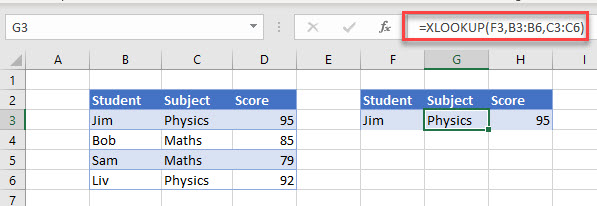1. Make these formulas visible on the Excel screen by pressing CTRL + ` and then, highlighting the formulas, use CTRL + C to copy them.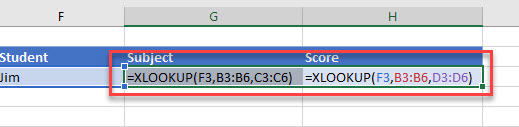1. Then switch to the Google spreadsheet and paste them where you want using CTRL + V.
2. However, while the formula pastes perfectly, an error occurs! This is because the XLOOKUP Function is not available in Google Sheets.